Courses
Courses for Kids
Free study material
Free LIVE classes
More

# Length Units## What are the Lengths of Units?

The term "length" refers to the size of an object or the distance between two points. The length of an object or the distance between two places is measured in length. It is used to determine the size of an object or the distance between two points.

In order to figure out what the basic length units are, we discover that there isn't one. This is because many measurement methods or systems are currently in use all over the world. However, the most commonly used length units today are US customary units and metric units, which include both SI and non-SI units. In addition, some countries continue to utilise British Imperial units. In this blog post, we'll be running through just a few of the most common units of measurement!

## Units of Measurement List

There are two types of units of measurement:

1. Non-standard unit

2. Standard unit

### Non-Standard Unit

• Non Standard unit is one unit from units of measurement list. Children in Foundation Stage learn about measuring without needing to read any scales by using non-standard units.

• The objective of non-standard measures is to focus the child on the concept of heavier, lighter, longer, shorter, etc. before they proceed onto the next step of measuring using standard units. Reading scales of any kind is a difficult ability in and of itself.

• For example, in order to measure height, handspan or foot is used but all people don’t have equal length of hand or foot this implies getting a standard unit of measurement.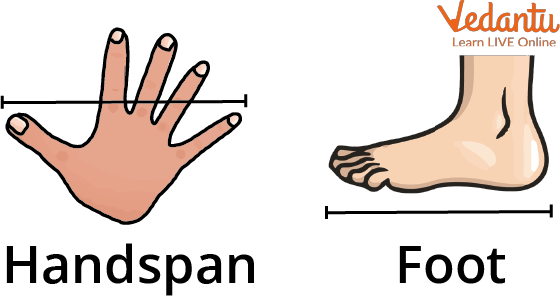Non-standard Unit

### Standard Unit

• A common unit of measurement is a quantifiable language that makes the relationship between the item and the measurement clear to all parties.

• In the US, it is measured in inches, feet, and pounds; in the metric system, it is measured in centimetres, metres, and kilograms.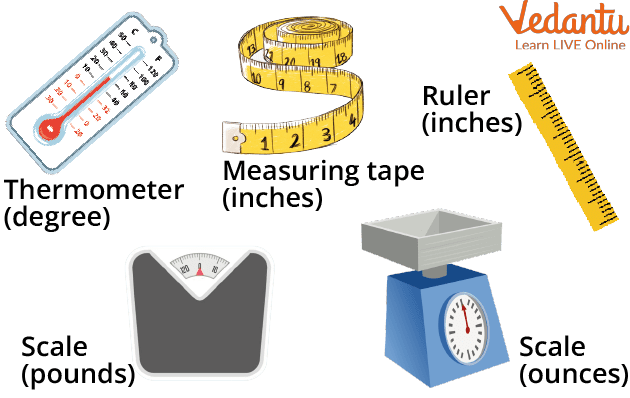Standard Unit

• For example, a ruler (used to measure height), measuring tape (used to measure length), scale (used for weight measurement), thermometer (temperature measurement), etc all are used to measure different types of things.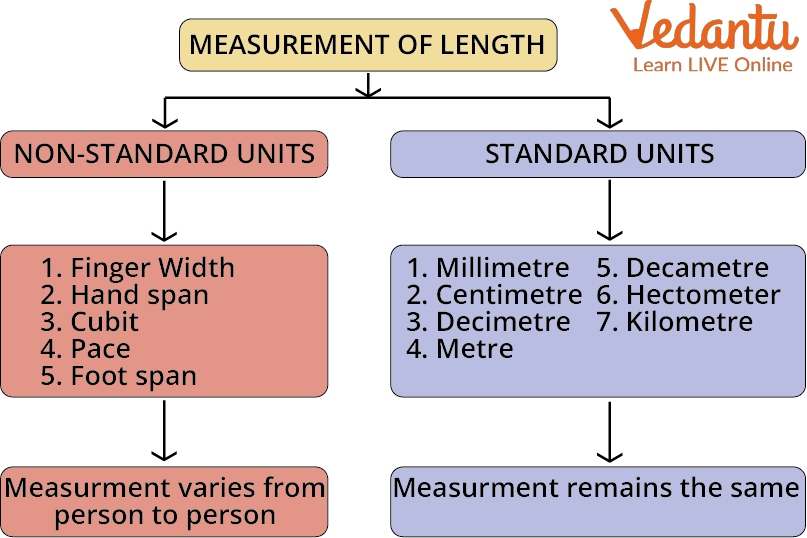Non-standard Unit and Standard Unit

## SI Unit of Length

The SI unit of length in the metric system is the metre.

The following are some of the other length units:

• Kilometre

• Hectometer

• Decameter

• Nanometer

• Millimetre

• Centimetre

• Decimeter

## Tools for Measuring Length

Tools help to get accurate measurements and this is the same for everyone at any place. A few tools that use for measuring length are:

• Ruler

• Scales

• Measuring tape

• Thermometer

• Protractors

## Order of Measurement

Small lengths (i.e. distances) are measured in cm and mm, while long lengths (i.e. distances) are measured in m and km. The 'Metre,' abbreviated as 'm,' is the standard unit of length.

One metre of length is divided into 100 equal parts. Each division is called a centimetre and is denoted by the letter 'cm.' As a result, 1 metre equals 100 centimetres.

The kilometre is the unit of measurement for the longest distance. One kilometre is divided into 1000 equal parts. A metre is the unit of measurement for each division. As a result; 1 kilometre equals 1000 metres.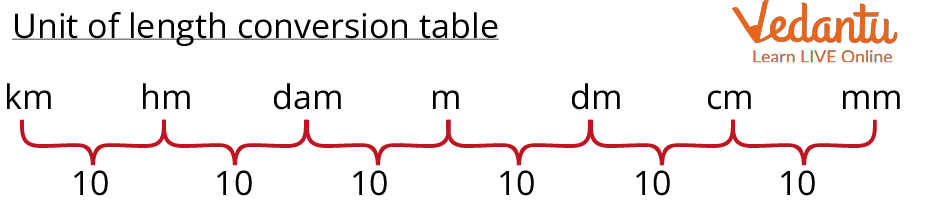Order of Measurement

Units of length and their counterparts, according to the length conversion charts, are:

1000 m = 1 kilometre (km) = 10 Hectometres (hm)

10 Decametres (dcm) = 100 Metres = 1 Hectometre (hm)

1 Metre (m) = 10 Decimetres (dm) = 100 Centimetres (cm) = 1000 Millimetres (mm)

10 Centimetres = 1 Decimetre (dm) (cm)

1 decimetre = 0.1 Metre

1 Centimetre (cm) = 10 Millimetres (mm) = 0.01 Metre

1 Millimetre = 0.001 Metre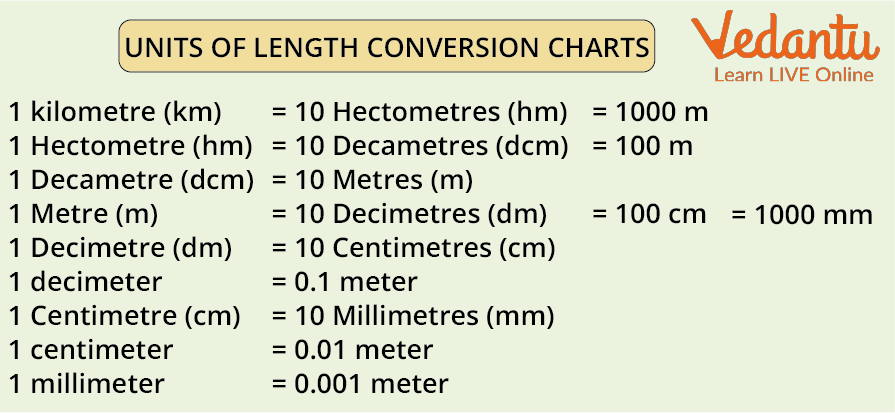Units of Length

## Metric System

A metric system is a unit of measurement for distance, length, volume, weight, and temperature. There are many different types of metric systems. It is built on three fundamental units that can be used to measure practically anything in the world.

Kg- kilogram, used to measure the mass

S- second, used to measure time

M- metre, used to measure the length

L-litre used to measure the length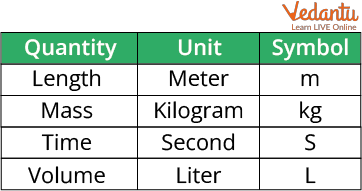Basic SI unit

## Types of Metric Systems

There are many different types of metric systems mentioned below;

1. SI System

The SI Units (Standard International System of Units) define the metre, kilogram, and second correctly.

2. CGS System

The CGS system of units is another system of expressing units in terms of length, weight, distance, and time.

3. Metric Units Smallest to Largest

Order of metric units smallest to largest: Nanometer, Millimetre, Decimeter, Metre, Decameter, Hectometer, Kilometre

## Summary

Length measurement is used to determine an object's length or the separation between two locations. It is employed to calculate an object's size or the separation between two spots. US customary units and metric units, which comprise both SI and non-SI units, are currently the most widely used length units. Additionally, some nations still make use of British Imperial units. The yard is the basic unit of length in both the Imperial and United States customary systems. In 1799, the metric system was created. The metric system of units is consistent and logical. This is a big benefit for usage in the home, in education, in business, and in science.

Last updated date: 16th Sep 2023
Total views: 113.7k
Views today: 1.13k

## FAQs on Length Units

1. What are 3 common units for length?

Distance measures length. For example, the distance of a road is how long the road is. In the metric system of measurement, the most common units of distance are millimeters, centimeters, meters, and kilometers.

2. What is the metric system based on? In a metric system, what are the basic units for length, weight, and volume?

The metric system of units is reliable and logical. In other simple words, because it is decimal, computations are simple and it fits together quite nicely. For use in the home, in education, in business, and in science, this is a significant benefit.

The metre and kilogram, introduced by France in 1799, are the foundations of the metric system, and a decimal-based system of measurement. All units are "decimal-based," which means they are based on powers of 10.

The base units for length, weight, and volume in the metric system are metres, grams, and litres, respectively.

3. Why is the metric system important?

A standardised approach that enables international scientists to communicate and compare notes is necessary. Without a standard, they would waste time transferring measurements from one system of measurement to another, and accuracy would decrease.